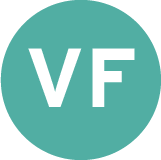Close

# PresentationOpening the black box of mathematics for CG [Short Course]
Coordinator
Lecturers
Event Type
Course
Hybrid FormatsRegistration CategoriesTime
Location
DescriptionMathematics is recognized as common basis for CG technology, but sometimes used as a black box in a CG software tool. We expect better understanding of maths will conduce to not only better CG tools, but also innovative ideas for a future production pipeline. The goal of this course is to pull the trigger for the graphics people to know more about usefulness and fun of the maths behind the scenes.

We therefore select a few typical CG topics ranging from elementary to standard levels, so that the course attendees can easily access the course content. We don’t assume that the attendees have familiarity with highly advanced mathematics. Elements of linear algebra and calculus at undergraduate level would be enough.

More specifically, we start with homogeneous coordinates, affine transformations and quaternions. These are common and basic mathematical concepts for CG. Matrix exponential and logarithm are then discussed for curve/surface editing, deformation and animation of geometric objects. Eigenvalues and eigenvectors are also well known mathematical concepts, yet appear with different faces in various graphics applications. In this course we intend to give a unified mathematical scope of these concepts. The corresponding graphics topics then include principal curvatures in geometry, PCA and Bayesian Inference in statistical approaches for animation, and Spherical Harmonics for image-based rendering. As an advanced topic, we briefly describe the mathematical concept called duality. Dual quaternion and theory of distribution will then be explained as mathematical basis of interpolation techniques for computer animation.

A unique feature of this course is that we demonstrate most of the mathematical concepts without rigorous formulation, while first showing their graphics applications. We expect this makes it easy to understand the mathematics mentioned above and to open the door for more advanced mathematical approaches.
CoordinatorLecturers